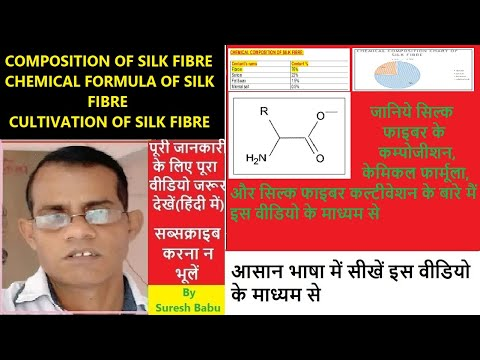## Friday, October 19, 2018

### YARN COUNT CONVERSION FROM ONE TO ANOTHER SYSTEM, YARN COUNT CONVERSION TABLE, TABLE OF YARN COUNT CALCULATION FORMULA

CONVERSION OF YARN COUNT FROM ONE SYSTEM TO ANOTHER SYSTEM:

The relation between New English count ( Ne) and denier:
The relation between new English count and denier can be derived as follows:
Suppose the new English count of yarn is C and denier is D.
As per the definition of new English count

The relation between new English count (Ne) and Tex:

The relation between new English count and tex can be derived as follows:
Suppose that Ne of yarn = C, and tex = T
As per the definition of new English count

The relation between Tex and Denier:

The relation between tex and denier can be derived as follows:
Suppose that tex of yarn is T, and denier is D

The relation between new English count (Ne) and the new metric count (Nm):

The relation between Ne and Nm can be derived as follows:
Suppose new English count = Ne, and new metric count = Nm

The relation between new English count (Ne) and linen count(lea):

The relation between new English count Ne and linen count can be derived as follows:
Suppose that new English count of yarn is Ne and linen count is a lea.

The relation between new metric count (Nm) and linen count(lea):

The relation between new metric count (Nm) and linen count(lea) can be derived as follows:
Suppose that new metric count of yarn is Nm and linen count of yarn is Lea.

The relation between new English count (Ne) and worsted count:

The relation between new English count and worsted count can be derived as follows:
Suppose that new English count = Ne, and worsted count of yarn is Cwst.

RELATION BETWEEN NEW ENGLISH COUNT AND WOOLEN COUNT(ysw)

Suppose that the new English count of a yarn is Ne and woolen count ( YSW) is WYSW
According to the definition of new English count
The length of 1 pound of yarn = Ne x 840 yards
As per the definition of woolen count ( ysw), Number of hanks of 256 yards in a pound is called woolen count(ysw)

RELATION BETWEEN NEW ENGLISH COUNT AND WOLLEN COUNT (DEWSBURY)

Suppose that new English count of yarn is Ne and woolen count( Dewsbury) is Wdew .
According to the definition of new English count, number of hanks of 840 yards in 1 pound
Length of 1 pound of yarn         = Ne X 840 yards
Or length of 16 ounces ( ozs )   = Ne X 840 yards
According to woolen count ( Dewsbury) count
Woolen count (Dewsbury)  Wdew = length in yards in 1 ounce of
Yarn

RELATION BETWEEN WOOLEN COUNT ( YSW ) AND WOOLEN COUNT ( DEWSBURY)

Suppose that woolen count ( ysw) of a yarn is WYSW and woolen count (Dewsbury) is Wdews.

According to the definition of woolen count (ysw), number of hanks of 256 yard in 1 pound
Thus the length of 1 pound of yarn = WYSW x 256 yards
Length of   16 ounces (ozs)        = WYSW x 256 yards
Since Length of 1 ounce ( oz) of yarn in yards is called woolen count (Dewsbury)
Relation between New english count( Ne) and jute count ( lbs.) :

RELATION BETWEEN LINEN COUNT( LEA) AND NEW METRIC COUNT ( Nm)

YARN COUNT CONVERSION TABLE:

TABLE OF YARN COUNT CALCULATION FORMULA

You may also interested in following articles:

1.Good job done

2.Good job done

3.## Featured Post

### COMPOSITION OF SILK FIBRE l CHEMICAL FORMULA OF SILK FIBRE l CULTIVATION...